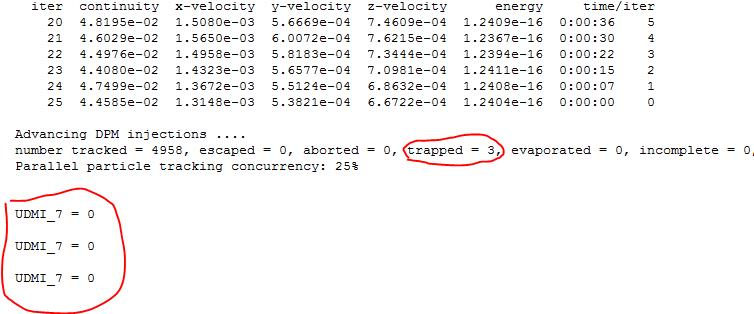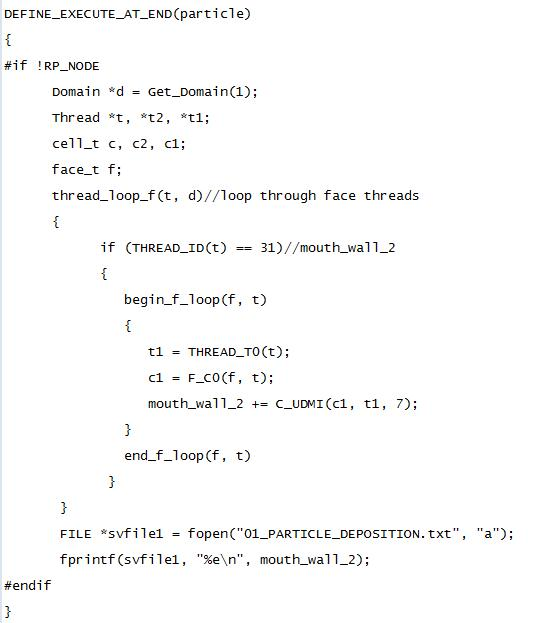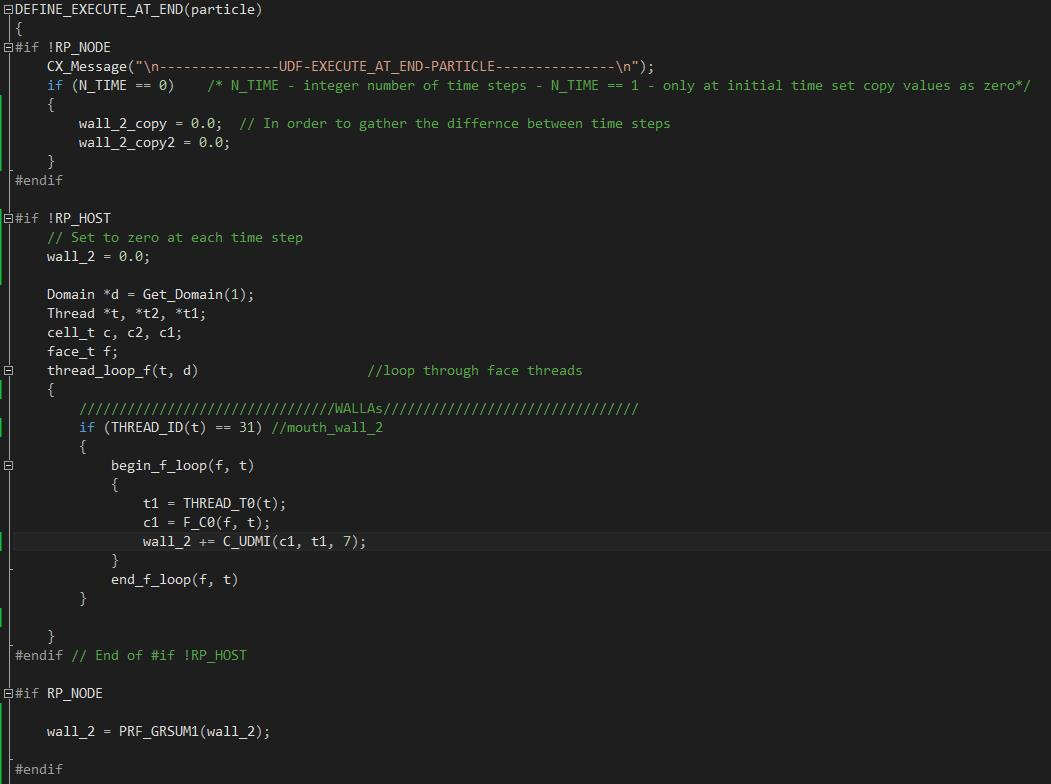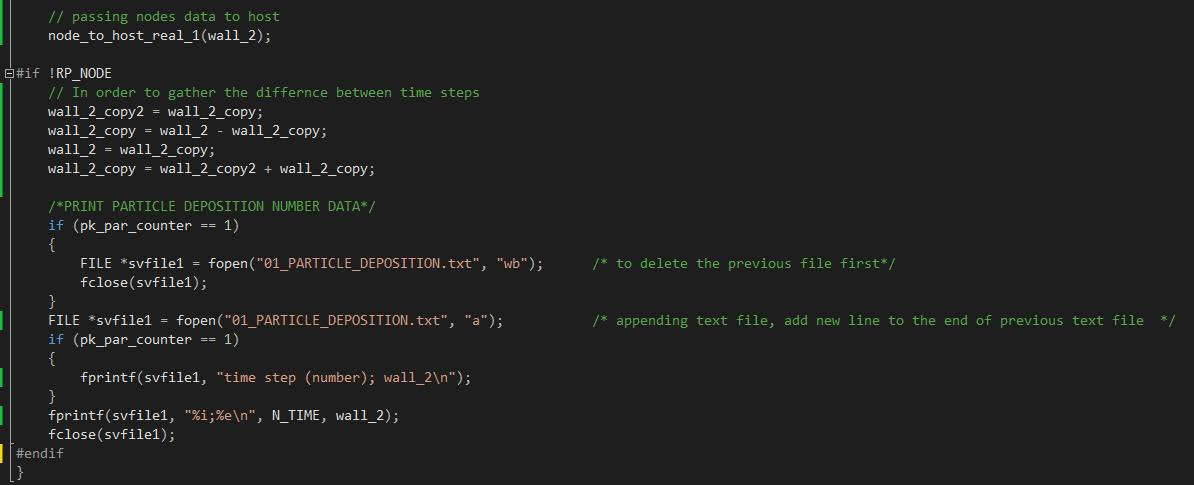## Fluids

•jianan.zhao
Subscriber

Hi everyone,

I'm trying to develop a DPM simulation using UDF to count particle deposition number on each wall region. The

deposition number is stored in a UDM.

The DPM was run in parallel using Hybrid method. However, when I try to write out the deposition number (UDM value)

to file, their values are all zero.

When I use Shared Memory method for DPM setup, the UDM values written to the file are correct, but simulation using

Shared Memory method is much slower than using Hybrid method.

So, my question is can I use hybrid method for DPM while write out the correct UDM data to file? Any comments and answers are appreciated. Thanks,

Jianan•jianan.zhao
Subscriber

I have attached the UDFs below. DEFINE_DPM_EROSION is developed to count for deposition number on each

cell surface and store the value in UDM. Then, DEFINE_EXECUTE_AT_END is used to caculate the deposition number on

each wall surface and write out the result in a txt file.

DEFINE_DPM_EROSION(particle_deposition_2, p, t, f, normal, alpha, Vmag, Mdot)

{

cell_t c1;

double A[ND_ND];

double area;

F_AREA(A, f, t);

area = NV_MAG(A);

c1 = F_C0(f, t);

C_UDMI(c1, t1, 7) = C_UDMI(c1, t1, 7) + 1.0;

C_UDMI(c1, t1,= C_UDMI(c1, t1, 7) / area;

CX_Message("UDMI_7 = %in", C_UDMI(c1, t1, 7));

} // end of DEFINE_DPM_EROSION•Rob
Ansys Employee

Break the problem down. The DPM part in the main solver is working & giving consistent results. What triggers a particle to register on UDM, and why is only part of the code parallelised?

Note, I'm not debugging the code: I'm asking questions so you can review what you've done.

•jianan.zhao
Subscriber

Hi Rwoolhou,

Here is the idea behind the code:

(1) Since I only need to count for the deposition number, particle doesn't have to register on UDM. So, basically UDMI is only a counter. Every time a particle hits a wall surface, DEFINE_DPM_EROSION is executed automatically, and then the value stored in UDMI plus 1.

(2) About parallel issue, do you suggest to parallelize  UDF DEFINE_DPM_EROSION, too? Is that the reason why Hybrid method cannot give the correct output?

I have read following in Fluent Customization Manual:

"Since all fluid variables needed for DPM models are held in data structures of the tracked particles, no special care is needed when using DPM UDFs in parallel ANSYS Fluent"

so I thought it is not necessary to parallelize UDF DEFINE_DPM_EROSION, and the code works when using Shared Memory method.

Anyway, I can give it a try and see if parallelization can solve the problem.

•jianan.zhao
Subscriber

So, I guess my question is where is UDMI data stored, in HOST or NODE? How does Hybrid and Shared Memory methods affect the data output?

Thank you.

Jianan

•jianan.zhao
Subscriber

I think I have found the problem.

The UDMI value is stored in NODEs, so that if using Hybrid method for DPM, one has to first gather data on NODEs and then pass the data to the HOST. After that HOST can process the data correctly, such as write to a local file. I have updated my UDF and posted here in case that anyone gets the same problem in the future.•DrAmine
Ansys Employee
Either host or node0 write data
•DrAmine
Ansys Employee
And nothing is stored on host. And the problem has nothing brto do with DPM parallel method. Thanks for sharing your solution.
Please mark this then as Is Solved.